# Summary Class notes - Survey Data Analysis

##### Course
- Survey Data Analysis
- Jean-Paul Fox
- 2016 - 2017
- Universiteit Utrecht
- Methodology and Statistics for the Behavioural, Biomedical and Social Sciences
200 Flashcards & Notes
1 Students

# Remember faster, study better. Scientifically proven.• ## 1476568800 Introduction in survey data analysis

• What is a survey?
A survey collects information about a well-defined population. This population need not necessarily consists of persons.

A survey collects information on only a small part of the population:
* a systematic method for data collection
* a sample from a finite population.
• What is a cencus or complete enumeration?
A cencus or complete enumeration is a way to obtain information about a population by collecting data about all its elements.
• What are three disadvantages of a census?
1. It is very expensive.
2. It is very time-consuming (this affects the timeliness of the results. Less timely information is less useful).
3. Large investigations increase the response burden on people.
• What is a sample?
A sample is a small part of the population that is used to collect information about a population.
• What is the object of a survey?
To construct quantitative descriptors of population (members)
• Where depends the data accuracy of a survey on?
On design and process
• What is a survey instrument?
Survey questionnaire, instructions, question, response scales.
• What is a population?
A population is a group of people whose beliefs, attitudes or activities are being studied.
• What is an observational unit or element?
An observational unit or element is a single measurement from the population.
• What is a sampling unit?
A sampling unit is a collection of units which can be sampled.
• What is a sampling frame?
A sampling frame is all sampling units in the population from which a sample can be taken.
• What is a sample?
A sample is the set of units that are drawn from the sampling frame
• What is needed in order to make inferences about a population based on a sample?
It is needed that the sample is selected using probability sampling. A random selection procedure uses an element of chance to determine which elements are selected, and which are not. If it is clear how this selection mechanism works and it is possible to compute the probabilities of being selected in the sample, survey results allow making reliable and precise statements about the population as a whole.
• What are the six steps of the survey process?
1. Survey design.
2. Data collection.
3. Data editing.
4. Nonresponse correction.
5. Analysis.
6. Publication.
• The first step in the survey proces is the survey design. What are five aspects that have to be addressed in defining a survey design?
1. Specifying the survey objectives: general questions.
2. The exact definition of the population that has to be investigated (the target population).
3. The specification of what has to be measured (the variables) and what has to be estimated (the population characteristics).
4. Where the sample is selected from (the sampling frame).
5. How the sample is selected (the sample design and the sample size).
• What is validity?
Validity is the extent to which a survey/instrument (questions) accurately measures the property it is supposed to measure.
• What are non-observational errors?
Non-observational errors occur when the sample data may not represent the entire population but only a part of it (selection bias).
• What are three categories of non-observational errors?
1. The sampling frame does not correspond correctly to the target population: over or under coverage.
2. Sampling error: since just a part of the population is observed (also known as margin of error).
• What are four sources of non-observational erros?
Observational errors is a class of errors introduced by..
1. the respondent (e.g. extreme response behaviour: socially desirable response behavior, overreporting/underreporting.
2. the interviewer (can influence respondent's responses)
3. the method of data collection
4. the measurement instrument: questions need to be clear.
• What is measurement error?
Measurement error is the difference between the survey response and the true response.
• Two types of inferences:
1) Of what can the characteristics of a respondent be inferenced?
2) What is inferenced from the characteristics of the sample?
1) The characteristics of a respondent can be inferenced from the respondents answers to the questions.
2) From the characteristics of the sample, the characteristics of the populations are inferenced.
• From measurement to representation: What kind of errors can be made in A - G?
A) Validity
B) Measurement error
C) Processing error
D) Coverage error
E) Sampling error
F) Nonresponse error
• From measurement to representation (see picture): What is measured on the right side and what on the left side? Fill in the dots
a) At the right side: .... is measured
At the left side: .... is measured

b) At the right side: .... measurements
At the left side: .... measurements
a) Right side (representation): who is measured
Left side (measurement): what is measured

b) Right side (representation): aggregate measurements
Left side (measurement): individual measurements
• What is a survey population?
A survey population is a collection of units to make quantitative statements about.
• What is a sampling frame?
A sampling frame is a list of all elements in the target population: a set of units with non-zero inclusion (selection) probabilitites.
• What is important for a sampling frame?
A sampling frame should be an accurate representation of the population. There is a risk of drawing wrong conclusions from the survey if the sample has been selected from a sampling frame that differs from the population.
• What are two possible problems that influence the representability of the sampling frame?
1. Undercoverage: this occurs if the target population contains elements that do not have a counterpart in the sampling frame : If the elements outside the sampling frame systematically differ from the elements in the sampling frame, estimates of population parameters may be seriously biased.
2. Overcoverage: when the sampling frame contains elements that do not belong to the target population. If such elements end up in the sample and their data are used in the analysis, estimates of population parameters may be affected.
• When is a sample representative?
A sample is said to be representative with respect to a variable if its relative distribution in the sample is equal to its relative distribution in the population.
• What is probability sampling?
Probability sampling is a stochastic method for selecting units from the sample
• What are design properties?
Design properties are a collection of methodological aspects leading to sample selection.
• What is an estimator?
An estimator is a function of sample data to produce an estimate of a population quantity.
• What is an estimate?
An estimate is a realization of the estimator for the particular considered sample (sample value, statistic).
• What are characteristics of a probability sample?
1. Each population unit (respondent) has a non-zero probability of being selected.
﻿2. The selection probability is known for elements in the sample (validity).
3. Pairs have a non-zero probability of being selected.
4. The selection probability for pairs is known for elements in the sample (accuracy).
• What is the sampling fraction of the elements in equal probability sampling?
For equal probability sampling is the sampling fraction: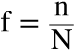• How can sampling weights be calculated?
The formula for a sampling weight is: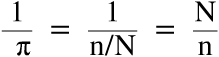• What is the formula for a weighted meaurement or weighted observation?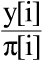• What is the selection probability for each unit in the population for an equal probability sampling with replacement when n elements are sampled out N?
For a population of size N, the selection probability is n/N
• What are disadvantages of sampling with replacement compared to sampling without replacement?
Sampling with replacement has lower precision than without replacement.
Sampling with replacement is inconvenient and not the standard.
• What is the selection probability for each unit in the population for an equal probability sampling without replacement when n elements are sampled out N?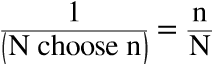• What are properties of estimators?
Name four properties.
Properties of the estimator are characteristics of the sampling distribution of the estimator.

1. Expectation
2. Variance (precision)
3. Bias
4. Mean squared error.
• What is the expectation of an estimator?
The expectation is the (weighted) average of all estimates, given all possible samples.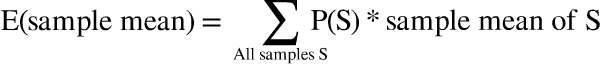• What is the bias of an estimator?
Bias is the difference between the expected and population value.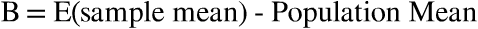• What is a sampling design?
A sampling design p assigns probabilities to each sample a.
• What is the variance of an estimator?
Variance is the discrepancy between sample realization and expectation.
• What is the mean squared error of an estimator?
The mean squared error is the discrepancy between sample relatization and the population value (is the variance of the estimator + the bias of the estimator squared).
• How is the sampling design connected to an estimator?
This summary. +380.000 other summaries. A unique study tool. A rehearsal system for this summary. Studycoaching with videos.

What is the effect of a stratified sample on p-values ignoring the survey design and what is the effect of a cluster sample?
p-values ignoring stratification will be too large: stratification often leads to more precise estimator.

p-values ignoring clusterig will be too small: clustering often leads to less precise estimators.
Where is the uncertainty based on in a design-based approach to sampling?
The randomization distribution defines the distribution of possible samples from the population, which defines the uncertainty. The uncertainty is based on the fact that some samples are more likely to be observed than others (more likely: smaller SE, less likely: larger SE).
What are five disadvantages of model-based inference over design-based inference?
1. All models are simplifications, misspecified models inferences are worse than design-based.
2. Modelers do not believe in random sampling (it is not the basis for inference). --> the sampling distribution depends on survey outcomes.
3.  Impractical for large-scale surveys: building strong models, computational complexity of model fitting.
4. Challenge: how exactly to specify the model?
5. Pay attention to fit diagnostics.
What are two advantages of model-based inference over design-based inference?
1. Unified approach to inference
2. Connection to design-based inferences possible. Choose a model that incorporates design features (weighting, stratification, clustering).
What are four disadvantages of design-based inference over model-based inference?
1. The theory doesn't allow you to make small sample adjustments.
2. No theory for optimal estimation (it should be unbiased and it should give you realistic estimators, based on design. There is no additional tool to assess if it’s the optimal estimate in the sense that it leads to the smallest e.g. variance).
3. Variance computation under poststratification: Potentially, it is possible to not being able to compute the variance (when there are groups with zero observations after poststratification).

4. Systematic errors: difficulty in population predictions because systematic errors are made (e.g. non-response, under- or overcoverage).
What are two advantages of design-based inference over model-based inference?
1. The survey design features are taken into account.
2. Reliable inferences in large samples (does not have strong model assumptions).
What are five characteristics of a model-based approach?
1. Under the model, a joint distribution is assumed for the outcomes Y (which is a random variable).
2. Values for the finite population are one realization for the random variable (superpopulation model).
3. The joint distribution is the link between units in the sample and not in the sample.
4. Observations are used to predict the unobserved values.
5. The randomization distribution is not used.
What are four characteristics of the finite population sample?
1. Randomization theory / design-based approach to sampling.
2. The observations are fixed but unkown.
3. The random variables indicate which population units are in the sample.
4. The randomization distribution defines the distribution of the possible samples from the population.
What is the Wald test statistic?
See picture. A Wald test-statistic can be used in the case of complex surveys, because the variance of the estimator accounts for the complex design.
What is model-assisted estimation?
In model-assisted estimation, a population model motivates the form of the estimator, but inferences are based on the sampling design.